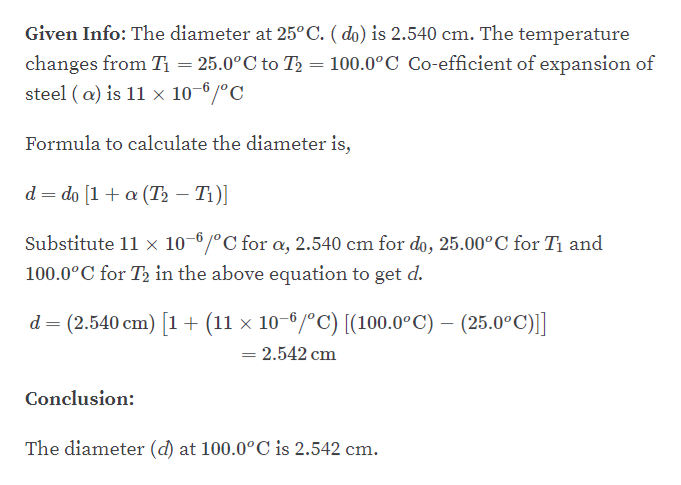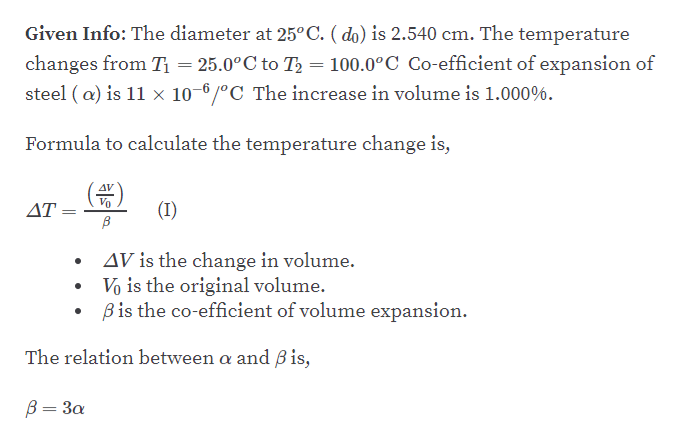# A spherical steel ball bearing has a diameter of 2.540 cm at 25.00°C. (a) What is its diameter when its temperature is raised to 100.0°C? (b) What temperature change is required to increase its volume by 1.000%?

Question
1 views

A spherical steel ball bearing has a diameter of 2.540 cm at 25.00°C. (a) What is its diameter when its temperature is raised to 100.0°C? (b) What temperature change is required to increase its volume by 1.000%?

check_circle

Step 1

Part A:help_outlineImage TranscriptioncloseGiven Info: The diameter at 25°C. ( do) is 2.540 cm. The temperature = 100.0°C Co-efficient of expansion of changes from T = 25.0°C to T steel ( a) is 11 x 10-0/°C Formula to calculate the diameter is, d = do [1+ a (T, – T1)] %3D Substitute 11 × 10-6/°C for a, 2.540 cm for do, 25.00°C for Tị and 100.0°C for T, in the above equation to get d. d = (2.540 cm) [1 + (11 × 10–6/ºC) [(100.0°C) – (25.0°C)] = 2.542 cm Conclusion: The diameter (d) at 100.0°C is 2.542 cm. fullscreen
Step 2

Part B:...help_outlineImage TranscriptioncloseGiven Info: The diameter at 25°C. ( do) is 2.540 cm. The temperature changes from T = 25.0°C to T2 = 100.0°C Co-efficient of expansion of steel ( a) is 11 × 10–6 /°C The increase in volume is 1.000%. Formula to calculate the temperature change is, AV (I) ΔΤ. AV is the change in volume. Vo is the original volume. Bis the co-efficient of volume expansion. The relation between a and B is, B= 3a fullscreen

### Want to see the full answer?

See Solution

#### Want to see this answer and more?

Solutions are written by subject experts who are available 24/7. Questions are typically answered within 1 hour.*

See Solution
*Response times may vary by subject and question.
Tagged in

### Other